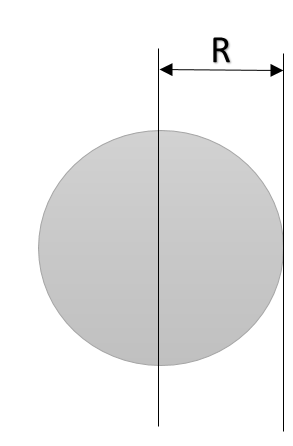Courses
Courses for Kids
Free study material
Free LIVE classes
MoreLIVE
Join Vedantu’s FREE Mastercalss

# The moment of inertia of a uniform solid sphere w.r.t tangent to its surface is:$\text{A.}\quad \dfrac25 MR^2$$\text{B.}\quad \dfrac75 MR^2$$\text{C.}\quad \dfrac23 MR^2$$\text{D.}\quad \dfrac53 MR^2$Verified
323.1k+ views
Hint: To find the moment of inertia of any shape about an unknown axis, first we need to know the moment of inertia of the shape about an axis either perpendicular to it or parallel to it. If we know the moment of inertia of a shape about an axis passing through the centre of mass of the body, then we can find the moment of inertia about any axis parallel to it by the use of parallel axis theorem.

Formula used:
${ I }_{ axis }={ I }_{ com }\ +\ Md^{ 2 }$ [ Statement of parallel axis theorem ]

Since every diameter of a sphere passes through its centre of mass, hence the moment of inertia of a sphere about its diameter is also the moment of inertia about its centre of mass. Now, to find the moment of inertia about its tangent, the distance between diameter and a tangent equals ‘R’.
Given, $I_{com} = \dfrac25 MR^2$and $d= R$, as we know, the moment of inertia of the solid sphere about its axis passing through its centre of mass is $\dfrac{2}{5}MR^2$.Now using parallel axis theorem:
$I_{axis} = I + MR^2$ ------ 1.
Hence $I$=$\dfrac{2}{5}MR^2$
$I_{axis} = \dfrac25 MR^2 + MR^2 = \dfrac 75 MR^2$,

So, the correct answer is “Option B”.

Note: While using the parallel axis theorem, one axis should always be the one passing through the centre of mass. Chance of mistakes is high if one avoids this information. Students are advised to learn the moment of inertia of commonly used shapes for example: Disc, sphere, etc. $\dfrac25MR^2$is the moment of inertia of a solid sphere about an axis passing through its centre of mass. One must not get confused with solid and hollow bodies either. The moment of inertia of a hollow sphere along an axis passing through its COM is $\dfrac 23MR^2$.
Last updated date: 20th Sep 2023
Total views: 323.1k
Views today: 3.23k